# Fibonacci Series in Java Using Recursion

Fibonacci series in Java
Write a Java program to print Fibonacci series up to a given number or create simple Java program to calculate Fibonacci number is common Java questions on fresher interview and homework. Fibonacci series is also a popular topic on various programming exercises in school and colleges. Fibonacci series is series of natural number where next number is equivalent to the sum of previous two number e.g. fn = fn-1 + fn-2. The first two numbers of Fibonacci series is always 1, 1. In this Java program example for Fibonacci series, we create a function to calculate Fibonacci number and then print those numbers on Java console. Another twist in this questions is that sometime interviewer asks to write a Java program for Fibonacci numbers using recursion, so it's better you prepare for both iterative and recursive version of Fibonacci number.

One more coding question which is quite popular is printing prime numbers in Java which I have discussed earlier. In this tutorial, we will see an example of both recursive and iterative algorithm for Fibonacci series in Java.

For more coding questions you can always look into Cracking the Code Interviews, one of the finest collections of code based questions from programming interviews. You will find data structure and algorithmic questions asked on companies like Amazon, Google, Microsoft, and Facebook on this book.

## Printing Fibonacci numbers in Java : Sample Code Example

Here is complete code example of printing Fibonacci Series in Java. Fibonacci series is calculated using both Iterative and recursive method and written in Java programming language. We have two functions in this example, fibonacci(int number)  and  fibonacci2(int number).The first one print Fibonacci series using recursion and the second one using for loop or iteration.

You can test this code on your computer as well. One you create your Java source file, just compile and run. It will ask you to enter the number till which you want to see the series. Once you enter then a number, it will print the Fibonacci series in the console.

## Java Program to print Fibonacci Series

```import java.util.Scanner;

/**
* Java program to calculate and print Fibonacci number using both recursion
* and Iteration.
* Fibonacci number is sum of previous two Fibonacci numbers fn= fn-1+ fn-2
* first 10 Fibonacci numbers are 1, 1, 2, 3, 5, 8, 13, 21, 34, 55
*
* @author Javin
*/
public class FibonacciCalculator {

public static void main(String args[]) {

//input to print Fibonacci series upto how many numbers
System.out.println("Enter number upto which Fibonacci series to print: ");
int number = new Scanner(System.in).nextInt();

System.out.println("Fibonacci series upto " + number +" numbers : ");
//printing Fibonacci series upto number
for(int i=1; i<=number; i++){
System.out.print(fibonacci2(i) +" ");
}

}

/*
* Java program for Fibonacci number using recursion.
* This program uses tail recursion to calculate Fibonacci number for a given number
* @return Fibonacci number
*/
public static int fibonacci(int number){
if(number == 1 || number == 2){
return 1;
}

return fibonacci(number-1) + fibonacci(number -2); //tail recursion
}

/*
* Java program to calculate Fibonacci number using loop or Iteration.
* @return Fibonacci number
*/
public static int fibonacci2(int number){
if(number == 1 || number == 2){
return 1;
}
int fibo1=1, fibo2=1, fibonacci=1;
for(int i= 3; i<= number; i++){

//Fibonacci number is sum of previous two Fibonacci number
fibonacci = fibo1 + fibo2;
fibo1 = fibo2;
fibo2 = fibonacci;

}
return fibonacci; //Fibonacci number

}

}

Output:
Enter number upto which Fibonacci series to print:
12
Fibonacci series upto 12 numbers :
1 1 2 3 5 8 13 21 34 55 89 144```

After asking to write simple Java program to print Fibonacci series and later asking for Fibonacci series using recursion, another important question interviewer ask is how do you improve your Fibonacci function both iterative and recursive one?

A technique called memoization can be used to drastically improve performance of method which calculates Fibonacci number. if you look at the method it repetitive creates same Fibonacci number e.g. In order to calculate 10th Fibonacci number function first create first 9 Fibonacci number, this could be very time consuming if you just increase the upper limit from 10 to 10K.

In memoization programming technique, result of earlier calculation is cached and reused. So you don't need to create same Fibonacci number if you already have calculated it. You can learn more about improving the performance of algorithms by reading a good book on data structure and algorithms e.g. Introduction to Algorithms by Thomas Cormen.

You can write code for Fibonacci series with memoization by just using a HashMap and checking if Fibonacci number for a corresponding number is already exits or not and calculate only if it doesn't exist.

### Fibonacci Number in Java with Memoization

Here is the code example of printing Fibonacci number with memoization technique :

```    /*
* Java Program to calculate Fibonacci numbers with memorization
* This is quite fast as compared to previous Fibonacci function
* especially for calculating factorial of large numbers.
*/
public static int improvedFibo(int number){
Integer fibonacci = cache.get(number);
if(fibonacci != null){
return fibonacci; //fibonacci number from cache
}
//fibonacci number not in cache, calculating it
fibonacci = fibonacci2(number);

//putting fibonacci number in cache for future request
cache.put(number, fibonacci);
return fibonacci;
}```

Here is diagram of how Fibonacci series will look like when you draw :

```//comparison of performance of Fibonacci number with memoization
int number = 100000000;
long startTime = System.nanoTime();
int result = fibonacci2(number); //fibonacci number with memoization
long elapsedTime = System.nanoTime() - startTime;
System.out.println("Time taken to calculate Fibonacci number upto 100M
without memorization:" + elapsedTime);

startTime = System.nanoTime();
result = improvedFibo(number); //Fibonacci number with memoization
elapsedTime = System.nanoTime() - startTime;
System.out.println("Time taken to calculate Fibonacci number upto 100M
with memorization:" + elapsedTime);

Output:
Time taken to calculate Fibonacci number upto 100M without memoization:149479613
Time taken to calculate Fibonacci number upto 100M with memoization:118972384```

Interesting point is that improved method only shows better performance for large numbers like 100M otherwise iterative version of Fibonacci method is faster. That could be explained by extra work done by improved method in terms of storing value in cache and getting it from there or am I missing something? For more such questions for interviews, you can refer book like Programming Interviews Exposed by Wrox publication.

That's all on writing Java program to calculate and print Fibonacci series. Fibonacci number is good question for programming exercise but when asked as question in Java interview you just need to be more detailed and precise about what you are doing.

Further Learning
The Coding Interview Bootcamp: Algorithms + Data Structures
Data Structures and Algorithms: Deep Dive Using Java
Algorithms and Data Structures - Part 1 and 2

Other Java programming exercise and homework:

1.I had a homework exercise to write a Java program to print Fibonacci series up-to 100. Can I use your Java program to print Fibonacci series, is using recursion in a homework or programming exercise is valid ?

1.Recursion is actually an academic technique rather than professional one. I have hardly see any production code which is written using recursin, until ofcourse laguage optimize them into tail recursive algorithm to prevent stackoverflowerror. For example, Scala is a tail recursive language but not Java.

2.I've used recursion many times as a professional.
as an example, whenever your data set is a graph or hierarchy, the total number of children for any node should be a recursive algorithm

2.Shouldn't that be called "memoization" ?

1.Yes, the technique which caches values of previously calculated fibonacci numbers is known as "memoization", and NOT "memorization" (with r). Though I agree, second one sounds more reasonable as it keeps values in memory, does any one knows why it's other way around?

3.The comments in your code are wrong : you are indeed using recursion, but not tail-recursion.

Tail recursion means that the last action performed by the method is directly returning the value from the recursive call, without modifying it.

In your example, your function calls itself recursively twice, before adding the two return values and returning the result. Hence, you aren't performing tail recursion.

A correct tail recursion implementation would be :

public static int tailRecursiveFibonacci(long number) {
// Bootstrap
return tailRecursiveFibonacci(3, 1, 1, number - 3);
}
private static int tailRecursiveFibonacci(long currentRank, int antePreviousResult, int previousResult, long howManyMoreRanks) {
final int resultForCurrentRank = antePreviousResult + previousResult;
if (howManyMoreRanks == 0) return resultForCurrentRank;
return tailRecursiveFibonacci(currentRank + 1, previousResult, resultForCurrentRank, howManyMoreRanks - 1);
}

1.Actually, you don't need the HowManyMoreRanks parameter:

public int fib2 (n){
return fib (n,1,1)
}

public int fib_internal2 (int n, int acc1, int acc2) {

if (n == 0 || n == 1)
return acc2
else
return fib_internal2 (n-1, acc2, acc1 + acc2)

}

4.There is overflow for 48th fibonacci number for int return type.

1.See other comment about recursion vs tail recursion. The tail recursive solution carries everything it needs with it rather than leaving it behind to be recombined when the stack is unwound, thus does not need multiple stack frames and won't cause a stack overflow.

5.Anonymous is correct.. this program will overflow the moment it crosses integer bounds.. i think we should use double as the datatype to initialize the variables.

1.Not double, but surely can use long for calculating fibonacci series of a large number. Double or double is used for floating point numbers, while IMHO fibonacci seems an integral algorithm.

6.Two words as string values are provided as input. The program should find and print the positions having matching alphabets as output.

Example input and output:

If the input is "engine angry", the output should be "2 3" (As n in second position and g in third position match)
If the input is "male level", the output should be "4" (As e in fourth position matches)

1.import java.util.Scanner;

public class Question
{
public static void main(String[] args)
{
System.out.println("Enter 1st String");

String str1=(new Scanner(System.in)).next();
System.out.println("Enter 2nd String");
String str2=(new Scanner(System.in)).next();

for(int i=0;i<str1.length();i++)
{
for(int j=0;j<str2.length();j++)
if(str1.charAt(i)==str2.charAt(j) && i==j)
System.out.println("Idex is"+(i+1));
}

}

}

7.import java.io.IOException;

public class buddyq {
public static void main(String[] args) throws NumberFormatException, IOException {
System.out.println("enter the number::::::::::");
System.out.println("fibinocii numbers are:::::");

int f1, f2=0, f3=1;
for(int i=1;i<=a;i++){
System.out.print(" \n"+f3+"\n ");
f1 = f2;
f2 = f3;
f3 = f1 + f2;
}
}

}

8.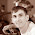"A technique called memorization can be used to drastically improve performance..." because formula F3=F2+F1 is very slow for large numbers (exponential complexity). Enjoy performance http://slorents.blogspot.com.au/2013/11/climb-ladder-with-n-step.html

1.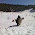Yes, it's called memoization (with the 'r'): Dynamic Programming.

9.The caching pattern is called "Memoization", not memorize.

http://en.wikipedia.org/wiki/Memoization

10.Here is a smart-alec implementation:

public class Fib
{
static final double a1 = (Math.sqrt(5) + 1)/2;
static final double a2 = (Math.sqrt(5) - 1)/2;
static final double sq5 = Math.sqrt(5);

public static double fib(int n)
{
double term2 = (n % 2 == 1) ? Math.pow(a2,n) : -Math.pow(a2,n);
return (Math.pow(a1,n) + term2)/sq5;
}

public static void main(String[] args)
{
if (args.length < 1)
{
System.err.println("Missing argument");
System.exit(1);
}

int n = 0;

try
{
n = Integer.parseInt(args);
}
catch (Exception e)
{
System.exit(1);
}

System.out.println("fib(" + n + ") = " + fib(n));
}
}

The reason I called it smart-alec is that it defeats the likely purpose of the interview challenge - demonstrate your understanding of recursion. Fibonacci sequence can be computed using a formula which you can derive by solving a characteristic equation, and the computation will outperform the recursive or iterative method for sufficiently large values of N.

11.Hi,
There is faults in your code examples shown here.
The Fibonacci numbers starts with a 0.
The first 21 Fibonacci numbers Fn for n = 0, 1, 2, ..., 20 are:
F0 F1 F2 F3 F4 F5 F6 F7 F8 F9 F10 F11 F12 F13 F14 F15 F16 F17 F18 F19 F20
0 1 1 2 3 5 8 13 21 34 55 89 144 233 377 610 987 1597 2584 4181 6765
Please see Wiki page:
http://en.wikipedia.org/wiki/Fibonacci_numbers

12.i have d ful corect program

13.If you were to write this program in answer to a request in an interview, be sure to be rejected, for several reasons:

1) Neither the iterative version nor the recursive one are correct. Both will silently overflow. Worst, your benchmark is completely broken. Since you do not use the result, the compiler could as well not calculate anything. This is an optimization the Java compiler is able to do (although it will not do it on the first pass). If you had simply printed the result to the console, it would have make this optimization impossible. And by the way, you should have noticed that the result of your program is 1819143227. Is this in the expected range?

2) As 'Anonymous' said, your recursive function is not tail recursive. But beside this, Java is not a true recursive language, so no simple recursive version will work above 50 to 100. To write a truly recursive version, one has to write all the necessary code to store intermediate calculations on the heap instead of on the stack. This is a good exercise.

3) Your example of memoization is wrong since it does absolutely nothing. You are calling the fibonacci2 function only once with a single value. Obviously, it is not in the cache. So the times should be equal. The difference you see is only due to the fact that you are calling the "non optimized" version first. Try to call the optimized version first and you'll get the inverse result.

4) The program should produce a collection of fibonacci numbers. This should be independent of what you intend to do with theses numbers (for example print them to the console as a string, with spaces as separators).

Here is an example that gives the correct result:

import java.math.BigInteger;
import java.util.stream.Collectors;
import java.util.stream.Stream;

public class Fibonacci {

private static long number = 1000;

public static void main(String[] args) {
Stream fiboStream = Stream.iterate(new Tuple<>(BigInteger.ONE, BigInteger.ONE), x -> new Tuple<>(x._2, x._1.add(x._2))).limit(number).map(x -> x._1);
String result = fiboStream.map(BigInteger::toString).collect(Collectors.joining(" "));
System.out.println(result);
}

static class Tuple {
public final T _1;
public final U _2;
public Tuple(T t, U u) {
this._1 = t;
this._2 = u;
}
}
}

1.Great, now we have Fibonacci series implemented using Java 8 lambdas and Streams :)

2.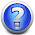except BigInteger::toString won't compile, toString is not a static method

14.What if I wanted to use non tail recursive to print Fibonacci numbers up to the value inputed?

15.What is recursive???

1.recursive means a function calls itself to calculate result. Many mathematical operations can be represented using recursion e.g. factorial of n is nothing but n* factorial(n-1), so you are calling same function again. This is recursion. In this program Fibonacci(n) = Fibonacci(n-1) + Fibonacci(n-2), that's why its recursive.

16.hi can i get the java program for this question:
Write a program to calculate the sum of integers 1 to n for a given number n
recursively. The program should contain a method to do the calculation and a main
method to test your method with the numbers 8 and 10.

17.public BigInteger fibonacci(BigInteger init, int index) {

BigInteger result = BigInteger.valueOf(1);

if (index != 0) {
index--;
result = fibonacci(init, index);
} else {
result = init;
}

return result;
}

This is the only approach in which you can get to 100 because the number is so big that int or long cannot hold it...

18.This post saved my ass. I used it to make my program for my Grade 12 Computer Science course for a project that was 2 weeks late. Of course, I had to make some alterations because I was putting it into a JFrame, and not just the normal output that is at the bottom when you normally run a program. But you really saved me here. Keep up the good work. Side note: It's an online class, and the content is not very descriptive, so I often have to teach myself. Recursion was particularly gruesome to find an explanation for that made sense to me.

19.class fib_recursive {

static void fib(int prevPrev, int prev, int limit){
int next = prevPrev + prev ;
System.out.println(next);
if(next > limit)
return ;

fib(prev,next,limit);
}

public static void main(String args[]) {

fib(0,1,30) ;

}
}

20.import java.util.*;
class FibSeries
{
public static void main(String[] args)
{
Scanner s=new Scanner(System.in);
System.out.println("Enter the value of n");
int n=s.nextInt();
int a=-1,b=1,temp;
for (int i=0;i<n ;i++ )
{

temp=b;

b=a+b;
a=temp;

System.out.println(b);
}
}
}

21.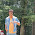As i have studied so far, Recursion means a function calls itself. Here in your program, the function is not calling itself. Then how is it recursion?
I dont think interviewer is going to satisfy with this code.

1.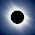Hello Arun, can you clarify which code? I can see it recursive?

22.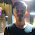package AllianzTechInterview;

public class FibonacciSeries {

public static void main(String[] args) {
// TODO Auto-generated method stub
int n1=0,n2=0,n3=1;

while(n3<=100)
{
n1=n2;
n2=n3;
n3=n1+n2;
System.out.println(n3);
}
}

}

1.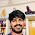int n1=0,n2=1,n3;
while(n3<=100){
n3=n1+n2;
System.out.println(n3);
n1=n2;
n2=n3;
}
it will give desire output febonacci:0 1 1 2 3.....

2.int n1=0,n2=1,n3;
while(n3<=100){
n3=n1+n2;
System.out.println(n3);
n1=n2;
n2=n3;
}
see it will work Exact febonacci:0 1 1 2 3 5......

23.static int fibonacci(int number, int fibonacciNumbers[]){
if(number == 0 || number == 1){
return 1;
}
assert fibonacciNumbers.length>number;
return fibonacciNumbers[number-2] + fibonacciNumbers[number-1];
}

public static void main(String a[])
{
int n = 50;
int[] fibonacciNumbers = new int[n];
for(int i=0; i<n; i++) {
fibonacciNumbers[i]= fibonacci(i,fibonacciNumbers);
System.out.print(fibonacciNumbers[i] +" ");
}

}

24.package interviewProgrammes;

import java.util.Scanner;

public class FibonocciSeries {

int[] intArray = null;

public void displayFiboSeries(int n) {

intArray = new int[n];

if(n>=2) {

intArray = 0;
intArray = 1;
}

if(n>2) {

for(int i = 2;i<n;i++) {

intArray[i] = intArray[i-1] + intArray[i-2];
}

}

for(int data:intArray) {
System.out.print(data + " ");
}

}

public static void main(String[] args) {

FibonocciSeries fibonocciSeries = new FibonocciSeries();

int num = new Scanner(System.in).nextInt();

fibonocciSeries.displayFiboSeries(num);

}

}

25.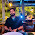import java.util.Scanner;

public class Fibonnic {

static int a=1;
static int b=1;
static int cont=1;

public static void main(String[] args) {
// TODO Auto-generated method stub
int num=new Scanner(System.in).nextInt();

System.out.println("Series.......");
for(int i=1;i<=num;i++)
{
System.out.println(fib(i));
}
}

private static int fib(int i) {

if(i==1 || i==2)
{
return 1;
}
else
{
cont =a+b;
a=b;
b=cont;
return cont;
}

}

}

26.import java.util.Scanner;
public class test1
{
public static void main(String args[])
{

int f1=0;
int f2=1;
int f3=f1+f2;
System.out.println("fabniconi serise is");
System.out.print(f1 + "\t" + f2 + "\t" + f3 + "\t");

for(int i=0;i<=100;i++)
{
f1=f2;
f2=f3;
f3=f1+f2;
System.out.print(f3 + "\t");
}

}
}

27.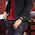import java.util.Scanner;
public class Fibonacci
{
public static void main(String args[])
{
System.out.print("Enter number till fibonacci series you want");
Scanner sc=new Scanner(System.in);
int number=sc.nextInt();
int a=1;
int b=1;

System.out.println(a);
for(int i=0;i<number;i++)
{

System.out.println(b);

b=a+b;
a=b-a;

}
}
}

28.public static void main(String[] args) {
int a=1; //starting variable number
int n=12; //number of series number to display
int b=0; //container variable

System.out.println(a);

for(int i=1;i<=n;i++) //for loop
{
a=a+b;
b=a-b;

System.out.println(a);
}
}

1.What is the output, very strange logic for fibonacci, never seen this before f(n) = f(n-1) + F(n-2) reads much better.

29.import java.util.Scanner;
public class fibo{

public static void main(String []args)
{
int a=0,b=0,c=1;
System.out.println("enter number");
Scanner sc=new Scanner(System.in);
int n=sc.nextInt();
for(int i=0;i<n;i++)
{
a=b;
b=c;
c=a+b;
System.out.println(c);
}

}
}

30.Hi what if we achieve it using array ?
I tried and it is working but would it be more efficient ?
I am not good with recursion :|